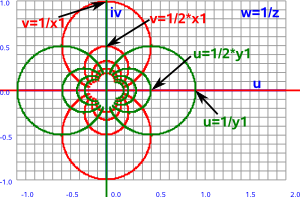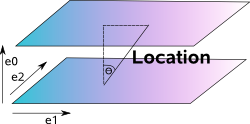# Maths - Möbius Transform

The Möbius transform can represent:

• Transforms in a hyperbolic plane: isometries that preserve the origin and orientation.
• Conformal map of the Riemann sphere to itself.
• Projective transforms.

Projective transforms extend the linear transforms to include things like reflection in a circle or sphere.

It is an important subject because its used when we are representing a 3D world on a 2D plane in a way that it appears to our eye. For instance: horizontal lines appear to meet at the horizon. For an application of this see OpenGL pages. Projective transforms and the Möbius transform are also important in physics for instance: Roger Penrose uses the stereographic projection to analyze relativity.

The equation for the Möbius transform is:Where:

• z = input (complex values)
• w = output (complex values)
• a,b,c,d = parameters (complex values)

Because this involves dividing by z we must add ∞ to the possible values of z and w to compactify the space, in other words, z and w are elements of the set (C U ∞).

Although projective transforms are not, in general, linear (they are a superset of linear transforms) they can be specified in a linear like form (matrix whose elements are complex numbers) by converting to homogeneous coordinates. Projective transforms are also a step in out searchTo convert to the linear equation we can start with the Möbius transform equation above and replace w and z with the ratios:

w = w1/w2
z = z1/z2

giving:multiplying top and bottom gives:equating the numerator and denominator of the equation gives:

w1 = a z1 + b z2
w2 = c z1 + d z2

which is a linear equation.

### Inverse Function

If we start with a simple case where a=d=0 and b=c=1 we get:

w=1/z

This inverse function is described on this page, we can see that vertical (red) and horizontal (green) lines in the z plane map to circles in the w plane:

z plane   w plane-->

w=1/zThe whole line in the z plane (including the point at infinity) is therefore mapped to the circle. The point at ∞ in z is mapped to 0 in the w plane. As long as the line does not go through the origin, in which case it would map to another line (infinite diameter circle), then we do not need to be concerned what the point (0,0) maps to.

If we now allow d to be non-zero we can see that this will just shift the line in the z-plane:

w=1/(z+d)

If we now allow c to scale we can see that this will scale the position on the line in the z-plane:

w=1/(cz+d)

If we now allow a and b to vary things get a bit more complicated but we can see that we can move the circle around the w plane.

## Algebraic Analysis

The equation:is the most general equation without any z² terms. This means that it can represent quantities that square to zero. Functions line exp() and sin() can be represented as a series and so can also take this form.

## Geometric InterpretationThe numerator and denominator can be thought of as linear functions in different planes. The ratio of these can be represented by a line which intersects these planes at an angle.

## Geometric Algebra Representation

So far we have represented the Möbius transform in homogeneous coordinates as a linear function which can be represented by matrices. Can we use geometric algebra instead? If we restrict the transforms in the plane to rotations then we can represent them using complex numbers.Roger Penrose - The Road to Reality: Partly a 'popular science' book as it tries to minimise the number of equations (Not that I'm complaining much his book 'Spinors and space-time' went over my head in the first few pages) it still has lots of interesting results that its difficult to find elsewhere.Spinors and Space-Time: Volume 1, Two-Spinor Calculus and Relativistic Fields (Cambridge Monographs on Mathematical Physics) by Roger Penrose and Wolfgang Rindler - This book is about the mathematics of special relativity, it very quickly goes over my head by I hope I will understand it one day.